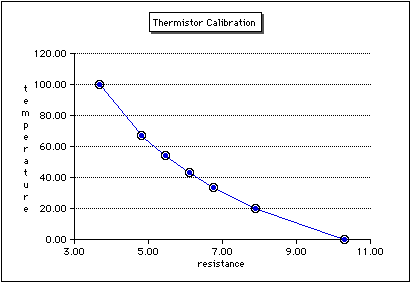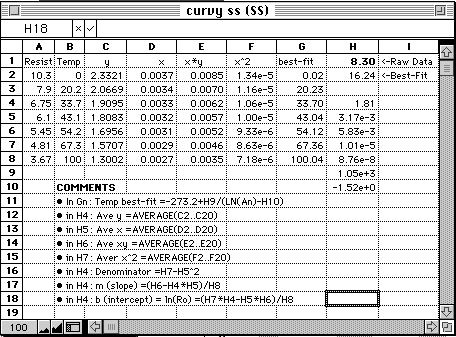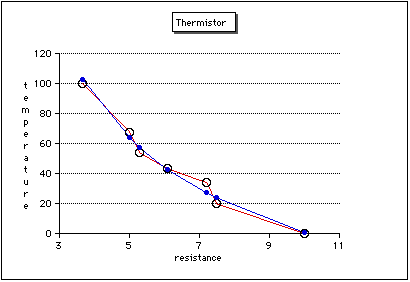# Hands-On-PhysicsHEAT & TEMPERATURE

## - Spreadsheets -

### What to do With Curvy Data

Fitting to a straight line is fine when the data should be straight (linear). But what about calibrating something like the thermistor that surely is not linear?Figure C10a
Non-linear Calibration Curve

Is there an alternative to looking up values on a graph? Is there a mathematical way of finding the best fit? The answer is yes, providing you know the equation of the curve. For instance, physics predicts that the resistance of a thermistor should obey the equation:

R = Ro * a / (T+To)
Here, Ro and a are the calibration constants like a and b in the straight-line fit. To is the ice temperature in the Kelvin scale, 273.2 °K. Once again, you don't have to understand the mathematics to use this equation. Here is a AppleWorks spreadsheet you can use with thermistor data. Just copy it into a AppleWorks spreadsheet and use it. Enter your calibration data into cells A2...B8. Enter a resistance reading in k in H1 and read the best fit temperature in °C in cell H2. The other cells in column H are used to calculate parts of the best-fit equation. The best-fit for temperatures for plotting the calibration curve are calculated in cells G2...G8.Figure C10b
Curvy Data Spreadsheet

The graph in figure C? shows the best-fit calculations lining up with the experimental measurements. Suppose the resistance measurements were not as carefully recorded and looked like this: 10, 7.5, 7.2, 6.1, 5.3, 5, and 3.67 K ohms. Then the calibration curve calculations would then be quite different than the experimental data.Figure C10c
Curve from Less Accurate Data

Previous Page || Up a Level || Index || Next Page